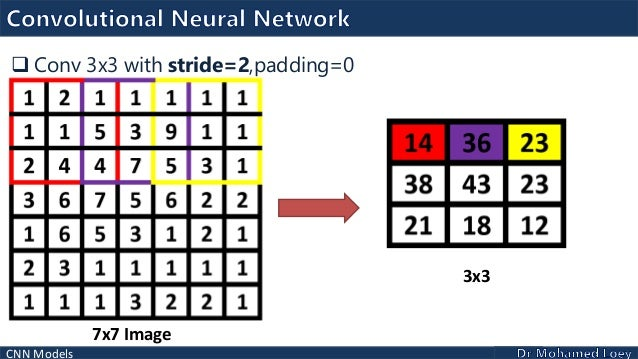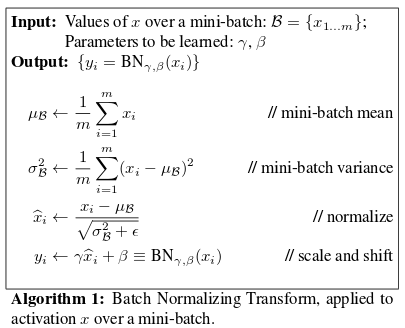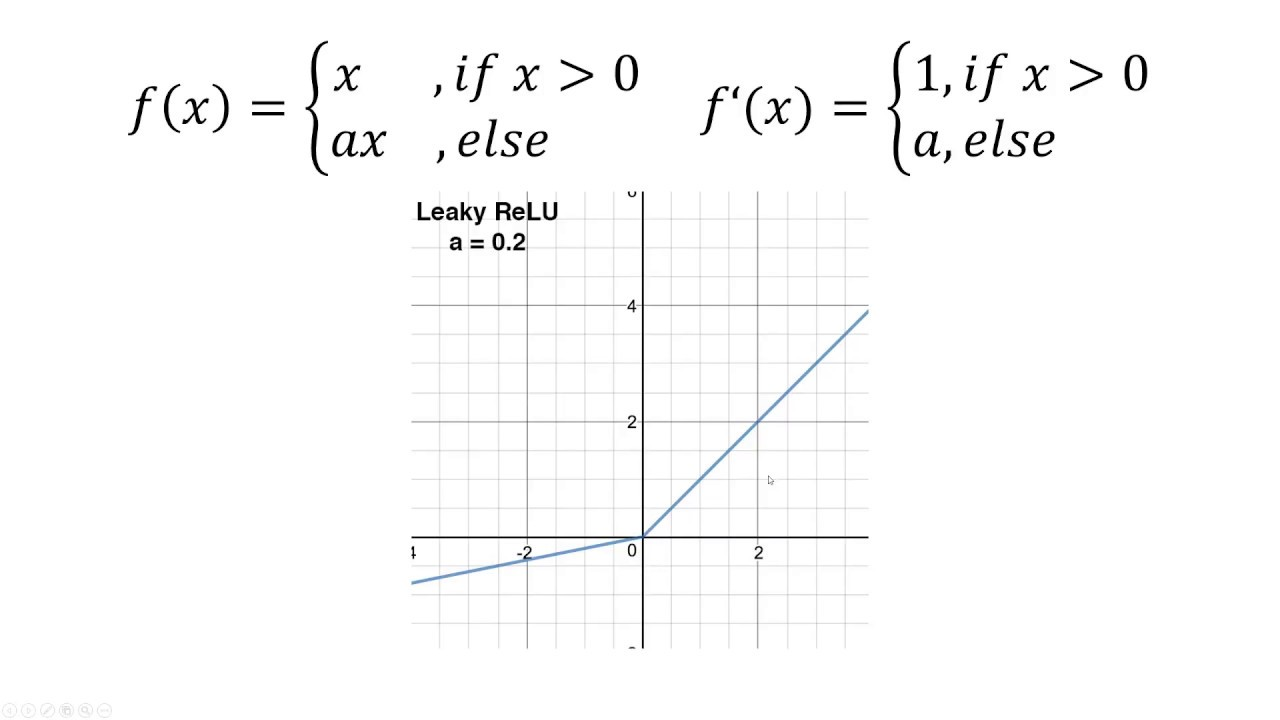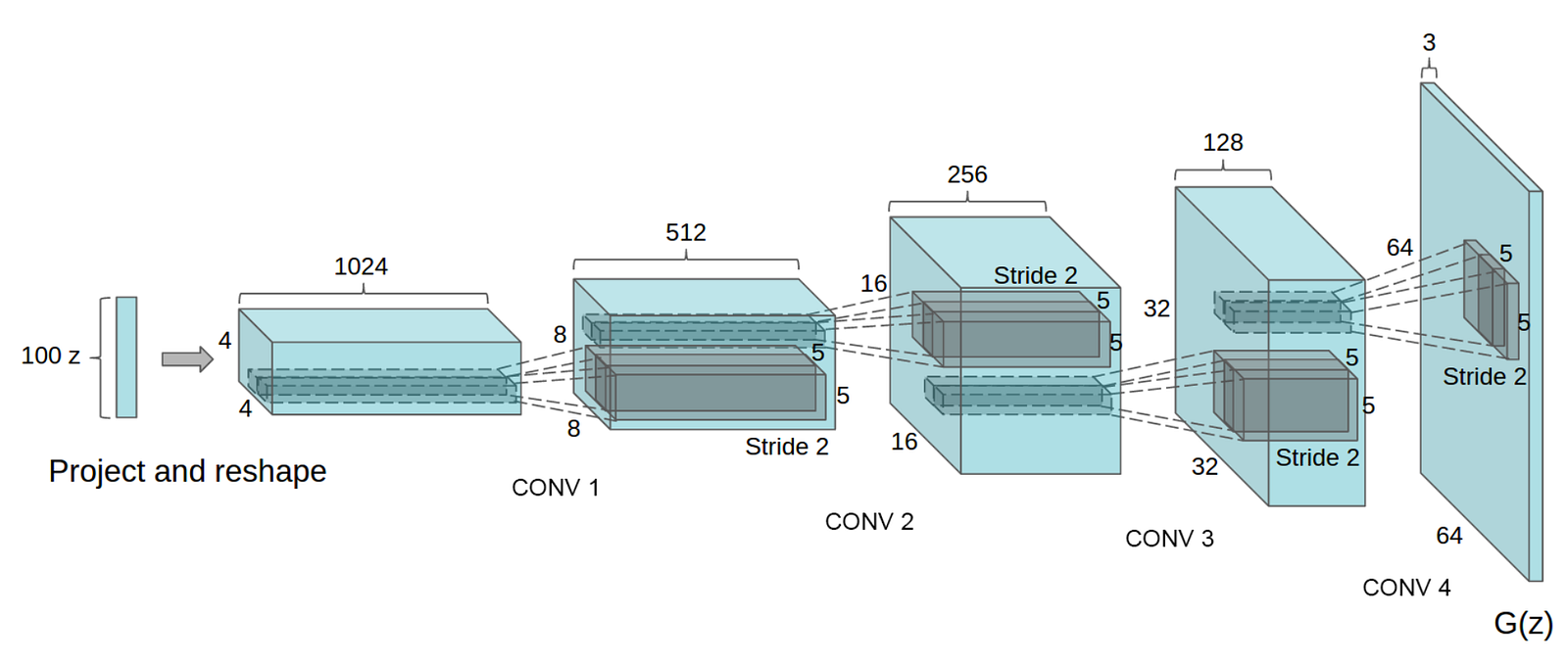Image credit: Gimages

# GAN 4

Deep Convolutional GAN

DGGAN is exactly the same as Linear GANs, excpet they use COnvolutional neural networks. As we all know CNNs are the best feature extractor for many kind of data like image, videos, audio, etc.

We will use Street View House (SVHN) Dataset and generate new home numbers using DCGAN.The model architecture will be the same, except the CNNs will be used in the Discriminator to classify images as real or fake. Generator will use a transpose convolutional layers to upsample/generate new image samples from a given latent vector z.

# Discriminator

In the original paper, no max-pooling layers are used with the CNN layers, rather a stride of 2 is used.Batch Normalization and Leaku ReLU are also used.Linear layers are connected at the end of flattened cnn layers and sigmoid activation is used to make the output in the range 0 to 1.

# Generator

In generator as we need to upsample a latent vector to an image of size [3, 32, 32], we use transposed convolutional layer with ReLU activation and Batch Normalization. Tanh activation in the output layer.# Let’s code the DCGAN

## Data

Pytorch have SVHN dataset built-in to the datset library, we will use that for the dataset.

import torch
from torchvision import datasets
from torchvision import transforms

transform = transforms.ToTensor()

batch_size=256,
shuffle=True)


We want to scale the images to have the value in the range -1 to 1.

def scale_img(x, feature_range=(-1, 1)):
min, max = feature_range
x = x * (max - min) + min
return x


## Discriminator

We will build a model with CNN and batch norm layers, it is a normal CNN classifier.

import torch.nn as nn
import torch.nn.functional as F

# function to return conv and batchnorm together
def conv(in_channels, out_channels, kernel_size, stride=2, padding=1, batch_norm=True):
layers = []
conv_layer = nn.Conv2d(in_channels, out_channels,
layers.append(conv_layer)

if batch_norm:
layers.append(nn.BatchNorm2d(out_channels))
return nn.Sequential(*layers)

class Discriminator(nn.Module):
def __init__(self, conv_dim=32):
super(Discriminator, self).__init__()
self.conv_dim = conv_dim
self.conv1 = conv(3, conv_dim, 4, batch_norm=False) #
self.conv2 = conv(conv_dim, conv_dim*2, 4)
self.conv3 = conv(conv_dim*2, conv_dim*4, 4)
self.fc = nn.Linear(conv_dim*4*4*4, 1)

def forward(self, x):
out = F.leaky_relu(self.conv1(x), 0.2)
out = F.leaky_relu(self.conv2(out), 0.2)
out = F.leaky_relu(self.conv3(out), 0.2)
out = out.view(-1, self.conv_dim*4*4*4)
out = self.fc(out)
return out


## Generator

Generator need transposed Convolutioanl layer with Batch Norm and relu to upsample the latent vector to a image sample.

def deconv(in_channels, out_channels, kernel_size, stride=2, padding=1, batch_norm=True):
layers = []
transpose_conv_layer = nn.ConvTranspose2d(in_channels, out_channels,
layers.append(transpose_conv_layer)
if batch_norm:
layers.append(nn.BatchNorm2d(out_channels))
return nn.Sequential(*layers)

class Generator(nn.Module):

def __init__(self, z_size, conv_dim=32):
super(Generator, self).__init__()
self.conv_dim = conv_dim
self.fc = nn.Linear(z_size, conv_dim*4*4*4)
self.t_conv1 = deconv(conv_dim*4, conv_dim*2, 4)
self.t_conv2 = deconv(conv_dim*2, conv_dim, 4)
self.t_conv3 = deconv(conv_dim, 3, 4, batch_norm=False)

def forward(self, x):
out = self.fc(x)
out = out.view(-1, self.conv_dim*4, 4, 4) # (
out = F.relu(self.t_conv1(out))
out = F.relu(self.t_conv2(out))
out = self.t_conv3(out)
out = F.tanh(out)
return out


## Building the models

conv_dim = 32
z_size = 100

D = Discriminator(conv_dim)
G = Generator(z_size=z_size, conv_dim=conv_dim)

print(D)
print()
print(G)

train_on_gpu = torch.cuda.is_available()

if train_on_gpu:
G.cuda()
D.cuda()
print('GPU available for training. Models moved to GPU')
else:
print('Training on CPU.')



Output :


Discriminator(
(conv1): Sequential(
(0): Conv2d(3, 32, kernel_size=(4, 4), stride=(2, 2), padding=(1, 1), bias=False)
)
(conv2): Sequential(
(0): Conv2d(32, 64, kernel_size=(4, 4), stride=(2, 2), padding=(1, 1), bias=False)
(1): BatchNorm2d(64, eps=1e-05, momentum=0.1, affine=True, track_running_stats=True)
)
(conv3): Sequential(
(0): Conv2d(64, 128, kernel_size=(4, 4), stride=(2, 2), padding=(1, 1), bias=False)
(1): BatchNorm2d(128, eps=1e-05, momentum=0.1, affine=True, track_running_stats=True)
)
(fc): Linear(in_features=2048, out_features=1, bias=True)
)

Generator(
(fc): Linear(in_features=100, out_features=2048, bias=True)
(t_conv1): Sequential(
(0): ConvTranspose2d(128, 64, kernel_size=(4, 4), stride=(2, 2), padding=(1, 1), bias=False)
(1): BatchNorm2d(64, eps=1e-05, momentum=0.1, affine=True, track_running_stats=True)
)
(t_conv2): Sequential(
(0): ConvTranspose2d(64, 32, kernel_size=(4, 4), stride=(2, 2), padding=(1, 1), bias=False)
(1): BatchNorm2d(32, eps=1e-05, momentum=0.1, affine=True, track_running_stats=True)
)
(t_conv3): Sequential(
(0): ConvTranspose2d(32, 3, kernel_size=(4, 4), stride=(2, 2), padding=(1, 1), bias=False)
)
)


## Loss

Loss and training loop is exactly same as Linear GANs. we scale the images in range -1 to 1 inside training loop. Check GAN2 and GAN3 is you dont understand about the loss and training loop.

def real_loss(D_out, smooth=False):
batch_size = D_out.size(0)
if smooth:
labels = torch.ones(batch_size)*0.9
else:
labels = torch.ones(batch_size)
if train_on_gpu:
labels = labels.cuda()
criterion = nn.BCEWithLogitsLoss()
loss = criterion(D_out.squeeze(), labels)
return loss

def fake_loss(D_out):
batch_size = D_out.size(0)
labels = torch.zeros(batch_size)
if train_on_gpu:
labels = labels.cuda()
criterion = nn.BCEWithLogitsLoss()

loss = criterion(D_out.squeeze(), labels)
return loss


## Optimizers

check this optimizer post by ruder


import torch.optim as optim

lr = 1e-4
beta1=0.5
beta2=0.999
d_optimizer = optim.Adam(D.parameters(), lr, [beta1, beta2])
g_optimizer = optim.Adam(G.parameters(), lr, [beta1, beta2])


## Training loop

num_epochs = 50
samples = []
losses = []

print_every = 300
sample_size=16
fixed_z = np.random.uniform(-1, 1, size=(sample_size, z_size))
fixed_z = torch.from_numpy(fixed_z).float()

for epoch in range(num_epochs):
for batch_i, (real_images, _) in enumerate(train_loader):
batch_size = real_images.size(0)
real_images = scale(real_images) # rescale image

if train_on_gpu:
real_images = real_images.cuda()

D_real = D(real_images)
d_real_loss = real_loss(D_real)

z = np.random.uniform(-1, 1, size=(batch_size, z_size))
z = torch.from_numpy(z).float()

if train_on_gpu:
z = z.cuda()
fake_images = G(z)
D_fake = D(fake_images)
d_fake_loss = fake_loss(D_fake)

d_loss = d_real_loss + d_fake_loss
d_loss.backward()
d_optimizer.step()

z = np.random.uniform(-1, 1, size=(batch_size, z_size))
z = torch.from_numpy(z).float()
if train_on_gpu:
z = z.cuda()
fake_images = G(z)
D_fake = D(fake_images)
g_loss = real_loss(D_fake)

g_loss.backward()
g_optimizer.step()

print('Epoch [{:5d}/{:5d}] | d_loss: {:6.4f} | g_loss: {:6.4f}'.format(
epoch+1, num_epochs, d_loss.item(), g_loss.item()))



Output:

Epoch [    1/   50] | d_loss: 1.3871 | g_loss: 0.7894
Epoch [    1/   50] | d_loss: 0.8700 | g_loss: 2.5015
Epoch [    2/   50] | d_loss: 1.0024 | g_loss: 1.4002
Epoch [    2/   50] | d_loss: 1.2057 | g_loss: 1.1445
Epoch [    3/   50] | d_loss: 0.9766 | g_loss: 1.0346
Epoch [    3/   50] | d_loss: 0.9508 | g_loss: 0.9849
Epoch [    4/   50] | d_loss: 1.0338 | g_loss: 1.2916
Epoch [    4/   50] | d_loss: 0.7476 | g_loss: 1.7354
Epoch [    5/   50] | d_loss: 0.8847 | g_loss: 1.9047
Epoch [    5/   50] | d_loss: 0.9131 | g_loss: 2.6848
Epoch [    6/   50] | d_loss: 0.3747 | g_loss: 2.0961
Epoch [    6/   50] | d_loss: 0.5761 | g_loss: 1.4796
Epoch [    7/   50] | d_loss: 1.0538 | g_loss: 2.5600
Epoch [    7/   50] | d_loss: 0.5655 | g_loss: 1.1675


## View generated Samples

def view_samples(epoch, samples):
fig, axes = plt.subplots(figsize=(16,4), nrows=2, ncols=8, sharey=True, sharex=True)
for ax, img in zip(axes.flatten(), samples[epoch]):
img = img.detach().cpu().numpy()
img = np.transpose(img, (1, 2, 0))
img = ((img +1)*255 / (2)).astype(np.uint8)
ax.xaxis.set_visible(False)
ax.yaxis.set_visible(False)
im = ax.imshow(img.reshape((32,32,3)))Training a DCGAN is same as the Linear/Vanilla GAN, DCGANs can extract more features in an image with the CNN and can help in generating the distributions well.

In the next post, we will look at Pix2Pix GAN and its applications.##### Shangeth Rajaa
###### Researcher at Vernacular.ai

Machine Learning Researcher at Vernacular.ai